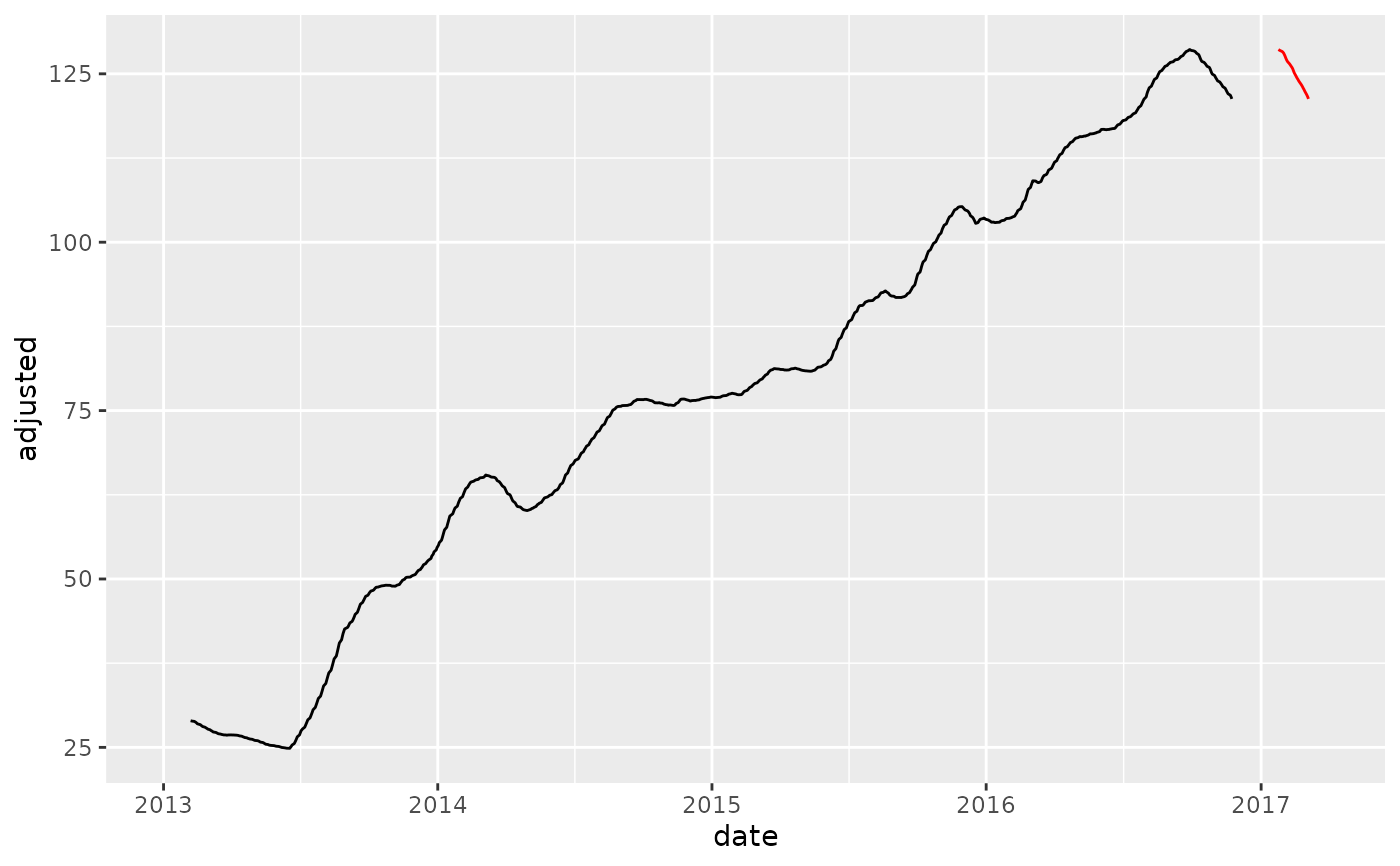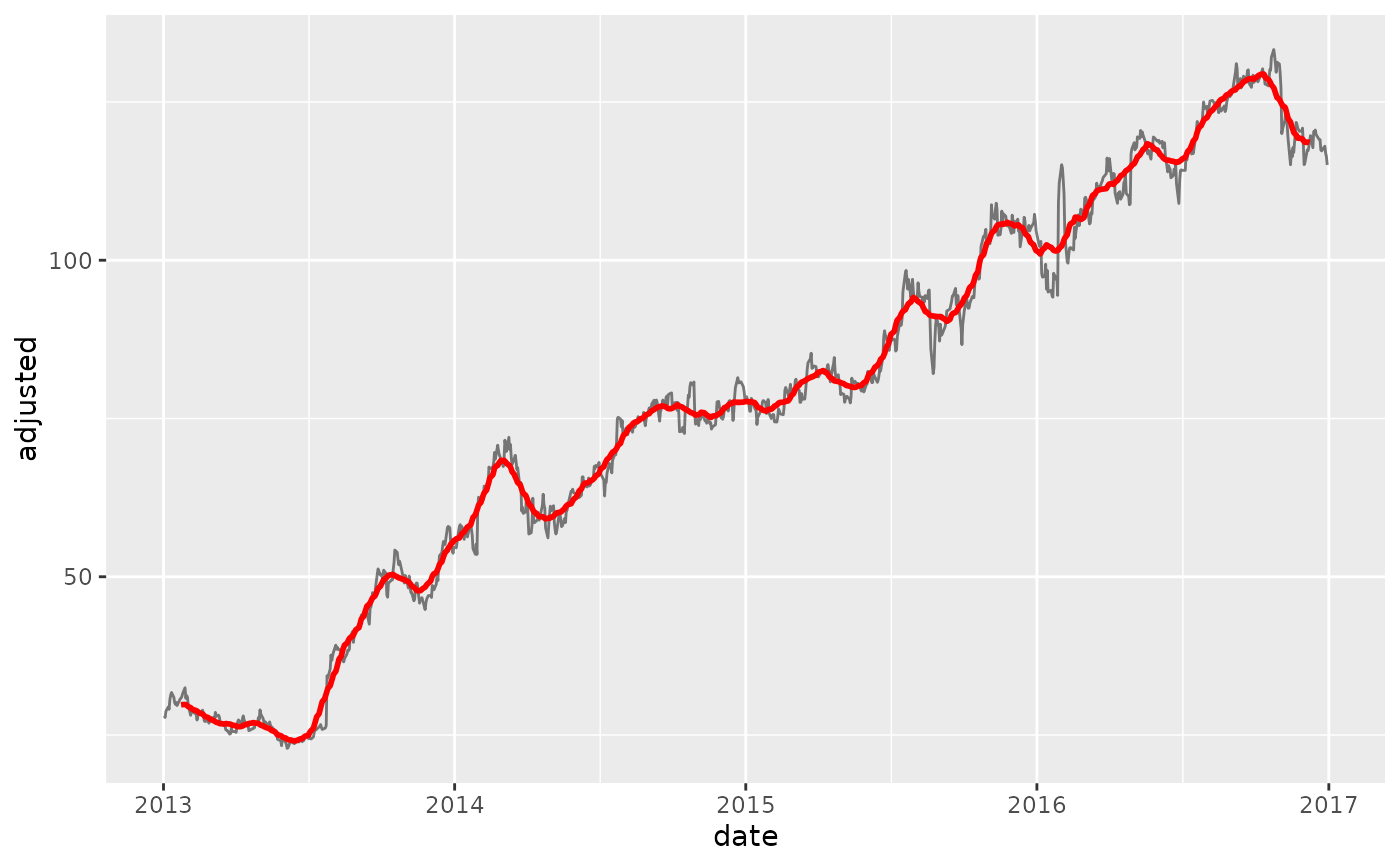step_slidify creates a a specification of a recipe step that will apply a function to one or more a Numeric column(s).

## Usage

step_slidify(
recipe,
...,
period,
.f,
align = c("center", "left", "right"),
partial = FALSE,
names = NULL,
role = "predictor",
trained = FALSE,
columns = NULL,
f_name = NULL,
skip = FALSE,
id = rand_id("slidify")
)

# S3 method for step_slidify
tidy(x, ...)

## Arguments

recipe

A recipe object. The step will be added to the sequence of operations for this recipe.

...

One or more numeric columns to be smoothed. See recipes::selections() for more details. For the tidy method, these are not currently used.

period

The number of periods to include in the local rolling window. This is effectively the "window size".

.f

A summary formula in one of the following formats:

• mean with no arguments

• function(x) mean(x, na.rm = TRUE)

• ~ mean(.x, na.rm = TRUE), it is converted to a function.

align

Rolling functions generate period - 1 fewer values than the incoming vector. Thus, the vector needs to be aligned. Alignment of the vector follows 3 types:

• Center: NA or .partial values are divided and added to the beginning and end of the series to "Center" the moving average. This is common for de-noising operations. See also [smooth_vec()] for LOESS without NA values.

• Left: NA or .partial values are added to the end to shift the series to the Left.

• Right: NA or .partial values are added to the beginning to shif the series to the Right. This is common in Financial Applications such as moving average cross-overs.

partial

Should the moving window be allowed to return partial (incomplete) windows instead of NA values. Set to FALSE by default, but can be switched to TRUE to remove NA's.

names

An optional character string that is the same length of the number of terms selected by terms. These will be the names of the new columns created by the step.

• If NULL, existing columns are transformed.

• If not NULL, new columns will be created.

role

For model terms created by this step, what analysis role should they be assigned?. By default, the function assumes that the new variable columns created by the original variables will be used as predictors in a model.

trained

A logical to indicate if the quantities for preprocessing have been estimated.

columns

A character string of variables that will be used as inputs. This field is a placeholder and will be populated once recipes::prep() is used.

f_name

A character string for the function being applied. This field is a placeholder and will be populated during the tidy() step.

skip

A logical. Should the step be skipped when the recipe is baked by bake.recipe()? While all operations are baked when prep.recipe() is run, some operations may not be able to be conducted on new data (e.g. processing the outcome variable(s)). Care should be taken when using skip = TRUE as it may affect the computations for subsequent operations.

id

A character string that is unique to this step to identify it.

x

A step_slidify object.

## Value

For step_slidify, an updated version of recipe with the new step added to the sequence of existing steps (if any). For the tidy method, a tibble with columns terms

(the selectors or variables selected), value (the feature names).

## Details

Alignment

Rolling functions generate period - 1 fewer values than the incoming vector. Thus, the vector needs to be aligned. Alignment of the vector follows 3 types:

• Center: NA or partial values are divided and added to the beginning and end of the series to "Center" the moving average. This is common for de-noising operations. See also [smooth_vec()] for LOESS without NA values.

• Left: NA or partial values are added to the end to shift the series to the Left.

• Right: NA or partial values are added to the beginning to shif the series to the Right. This is common in Financial Applications such as moving average cross-overs.

Partial Values

• The advantage to using partial values vs NA padding is that the series can be filled (good for time-series de-noising operations).

• The downside to partial values is that the partials can become less stable at the regions where incomplete windows are used.

If instability is not desirable for de-noising operations, a suitable alternative is step_smooth(), which implements local polynomial regression.

Time Series Analysis:

• Engineered Features: step_timeseries_signature(), step_holiday_signature(), step_fourier()

• Diffs & Lags step_diff(), recipes::step_lag()

• Smoothing: step_slidify(), step_smooth()

• Variance Reduction: step_box_cox()

• Imputation: step_ts_impute(), step_ts_clean()

• Padding: step_ts_pad()

Main Recipe Functions:

• recipes::recipe()

• recipes::prep()

• recipes::bake()

## Examples

library(recipes)
library(dplyr)
library(ggplot2)
library(tidyquant)
library(timetk)

# Training Data
FB_tbl <- FANG %>%
filter(symbol == "FB") %>%

# New Data - Make some fake new data next 90 time stamps
new_data <- FB_tbl %>%
tail(90) %>%
mutate(date = date %>% tk_make_future_timeseries(length_out = 90))

# OVERWRITE EXISTING COLUMNS -----

# Create a recipe object with a step_slidify
rec_ma_50 <- recipe(adjusted ~ ., data = FB_tbl) %>%
step_slidify(adjusted, period = 50, .f = ~ AVERAGE(.x))

# Bake the recipe object - Applies the Moving Average Transformation
training_data_baked <- bake(prep(rec_ma_50), FB_tbl)

# Apply to New Data
new_data_baked <- bake(prep(rec_ma_50), new_data)

# Visualize effect
training_data_baked %>%
geom_line() +
geom_line(color = "red", data = new_data_baked)
#> Warning: Removed 49 rows containing missing values (geom_line()).
#> Warning: Removed 49 rows containing missing values (geom_line()).# ---- NEW COLUMNS ----
# Use the names argument to create new columns instead of overwriting existing

rec_ma_30_names <- recipe(adjusted ~ ., data = FB_tbl) %>%
#> Warning: Using size aesthetic for lines was deprecated in ggplot2 3.4.0.
#> ℹ Please use linewidth instead.
#> Warning: Removed 29 rows containing missing values (geom_line()).